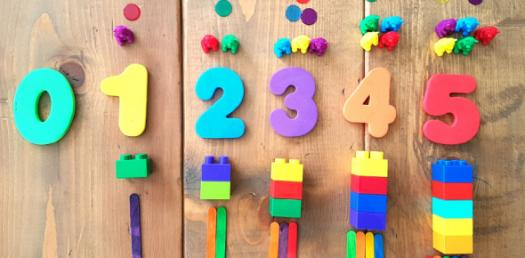# Math: Trivia Quiz On Number Concepts And Operations!

Approved & Edited by ProProfs Editorial Team
At ProProfs Quizzes, our dedicated in-house team of experts takes pride in their work. With a sharp eye for detail, they meticulously review each quiz. This ensures that every quiz, taken by over 100 million users, meets our standards of accuracy, clarity, and engagement.
| Written by Hatts
H
Hatts
Community Contributor
Quizzes Created: 12 | Total Attempts: 10,536
Questions: 8 | Attempts: 517Settings.

• 1.

### A + b = b +a is an example of which mathematical property?

• A.

Commutative Property (Order Property)

• B.

Associative Property (Grouping Property)

• C.

Distributive Property

A. Commutative Property (Order Property)
Explanation
The given equation, a + b = b + a, demonstrates the commutative property of addition. This property states that the order of adding two numbers does not affect the sum. In other words, when adding two numbers, it doesn't matter which number comes first, the result will remain the same.

Rate this question:

• 2.

### A + (b +c) = (a + b) + c is an example of which mathematical property?

• A.

Commutative Property (Order Property)

• B.

Associative Property (Grouping Property)

• C.

Distributive Property

B. Associative Property (Grouping Property)
Explanation
The given equation, a + (b + c) = (a + b) + c, demonstrates the Associative Property (Grouping Property) in mathematics. This property states that the grouping of numbers being added or multiplied does not affect the result. In this case, regardless of whether we first add b and c or a and b, the final sum will be the same. The equation shows that the addition of b and c is grouped together and then added to a, which is equivalent to adding a and b first and then adding c.

Rate this question:

• 3.

### A x (b + c) = (a x b) + (a x c) is an example of which mathematical property?

• A.

Commutative Property (Order Property)

• B.

Associative Property (Grouping Property)

• C.

Distributive Property

C. Distributive Property
Explanation
The given equation, a x (b + c) = (a x b) + (a x c), represents the Distributive Property. This property states that when multiplying a number by the sum of two other numbers, you can distribute the multiplication to each term inside the parentheses. In this case, the multiplication of a by the sum (b + c) is equal to the sum of the multiplication of a by b and the multiplication of a by c.

Rate this question:

• 4.

### What is the GCF/GCD of 16 and 24?

• A.

4

• B.

8

• C.

16

B. 8
Explanation
Find the GCF/GCD by listing all the factors and finding the largest one that is shared.

Rate this question:

• 5.

### What is the prime factorization of 120?

• A.

2 x 2 x 2 x3 x 5

• B.

10 x 12

• C.

2 x 2 x 5 x 6

A. 2 x 2 x 2 x3 x 5
Explanation
The prime factorization of a number involves finding the prime numbers that multiply together to give the original number. In this case, the prime factorization of 120 is 2 x 2 x 2 x 3 x 5. This means that 2, 3, and 5 are the prime numbers that, when multiplied together, equal 120.

Rate this question:

• 6.

### Multiples of 9 are what?

• A.

9,18,27...

• B.

3

A. 9,18,27...
Explanation
The given answer is incorrect. Multiples of 9 are numbers that can be obtained by multiplying 9 by any whole number. The correct answer should be "9, 18, 27, ..." as these are the numbers that can be obtained by multiplying 9 by 1, 2, 3, and so on.

Rate this question:

• 7.

### Find the LCM of 7 and 14

• A.

14

• B.

42

• C.

98

A. 14
Explanation
List the multiples of both nubmers until you find the lowest number shared by both.

Rate this question:

• 8.

### Find the LCD of 1/5 and 1/6

• A.

30

• B.

11

• C.

60Back to top